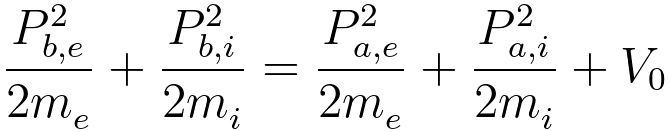Electron Wind Force
- Practical Electron Microscopy and Database -
- An Online Book -
Microanalysis | EM Book                                                                                   http://www.globalsino.com/EM/

=================================================================================

The concept of the ‘electron wind’ driving force was first introduced by Fiks and Huntington and Grone. In this case, an increasing current is applied to the material, very energetic electrons can be accelerated. Therefore, ions can be bombarded and displaced by these electrons, inducing momentum exchange, and thus displacement of material occurs. This results in the formation of voids in one place and hillocks in another, which often causes respectively open and short circuits and thus device failure. Furthermore, the electron wind force Fwind is normally larger than the electrostatic force Fstatic, and thus the momentum exchange between the electron wind and ions (or atoms) is normally the dominant mechanism for electromigration (EM).

In the electron wind theory, the number of collisions per unit time between the electrons and a moving atom is given by,
Nc = neveσe -------------------------- [1155a]
where,
ne -- the electron density,
ve-- the average velocity of the electrons,
σe -- the atom's intrinsic cross section for collision with the electrons.

Then, the the electron wind force can be given by,
Fwind = -|e|neλeσeξ -------------------------- [1155b]
where,
ξ -- the applied electric field,
λe -- the mean free path (= veτe),
τe -- the relaxation time.

The net force can also be given by,
F = Z*qξ = Z*qρJ ------------------------ [1155c]
where,
q -- the electronic charge,
ρ -- the electrical resistivity of the metal,
Z* -- the "effective" ion valence (normally far in excess of typical chemical valences),
J -- the macroscopic mass flux J (=Cv),
C -- the atomic concentration,
v -- the velocity.

The effect of the electron wind force in EM process is a very complex process; however, the EM process can be simplified. Let Pb,e and Pa,e be the momentum of the electron before and after collision, and Pb,i and Pa,i be the momentum of the ion before and after. The momentum and energy conservations give,
Pb,e + Pb,i = Pa,e + Pa,i ------------------------------------- [1155d]------------------------------ [1155e]
where,
me -- the mass of the electron,
mi -- the mass of the ion,
V0 -- the magnitude of the potential hole the ion is in before collision.

Note that, in order to move the ion, the electron’s kinetic energy must be greater than V0. The collision is elastic if V0 is equal to zero. An electron gains momentum if it moves against the electric field, while it loses a similar amount of momentum if it goes in the opposite direction.

 V. B. Fiks, 1959 Sou. Phys.-Solid State, 1, 14 (1959).
 H. B. Huntington and A. R. Crone, J. Phys. Chem. Solids, 20, 76 (1961).

=================================================================================